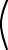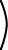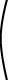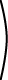det1 2 1 2= 1*2 - 2*(1) = 2 -2 = 0

Rank of a Matrix

The above matrix has a zero determinant and is therefore singular. It has no inverse. It has two identical rows. In other words, the rows are not independent. If one row is a multiple of another, then they are not independent, and the determinant is zero. (Equivalently: If one column is a multiple of another, then they are not independent, and the determinant is zero.)

The rank of a matrix is the maximum number of independent rows (or, the maximum number of independent columns). A square matrix An×n is non-singular only if its rank is equal to n.

QUESTION 15:

What is the rank of the following matrix?1 2 0 3 1 -2 3 0 0 0 4 8 2 4 0 6